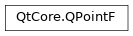# QPointF¶

The `QPointF` class defines a point in the plane using floating point precision. More## Detailed Description¶

A point is specified by a x coordinate and an y coordinate which can be accessed using the `x()` and `y()` functions. The coordinates of the point are specified using floating point numbers for accuracy. The `isNull()` function returns `true` if both x and y are set to 0.0. The coordinates can be set (or altered) using the `setX()` and `setY()` functions, or alternatively the `rx()` and `ry()` functions which return references to the coordinates (allowing direct manipulation).

Given a point p , the following statements are all equivalent:

```p = QPointF()

p.setX(p.x() + 1.0)
p += QPointF(1.0, 0.0)
#p.rx()++;
```

A `QPointF` object can also be used as a vector: Addition and subtraction are defined as for vectors (each component is added separately). A `QPointF` object can also be divided or multiplied by an `int` or a `qreal` .

In addition, the `QPointF` class provides a constructor converting a `QPoint` object into a `QPointF` object, and a corresponding `toPoint()` function which returns a `QPoint` copy of this point. Finally, `QPointF` objects can be streamed as well as compared.

`QPoint` `QPolygonF`

class PySide2.QtCore.QPointF

PySide2.QtCore.QPointF(p)

PySide2.QtCore.QPointF(QPointF)

PySide2.QtCore.QPointF(xpos, ypos)

param ypos:

float

param p:

`PySide2.QtCore.QPoint`

param xpos:

float

param QPointF:

`PySide2.QtCore.QPointF`

Constructs a null point, i.e. with coordinates (0.0, 0.0)

Constructs a copy of the given `point` .

Constructs a point with the given coordinates (`xpos` , `ypos` ).

PySide2.QtCore.QPointF.__reduce__()
Return type:

object

PySide2.QtCore.QPointF.__repr__()
Return type:

object

static PySide2.QtCore.QPointF.dotProduct(p1, p2)
Parameters:
Return type:

float

```QPointF p( 3.1, 7.1);
QPointF q(-1.0, 4.1);
int lengthSquared = QPointF::dotProduct(p, q);   // lengthSquared becomes 26.01
```

Returns the dot product of `p1` and `p2` .

PySide2.QtCore.QPointF.isNull()
Return type:

bool

Returns `true` if both the x and y coordinates are set to 0.0 (ignoring the sign); otherwise returns `false` .

PySide2.QtCore.QPointF.manhattanLength()
Return type:

float

Returns the sum of the absolute values of `x()` and `y()` , traditionally known as the “Manhattan length” of the vector from the origin to the point.

PySide2.QtCore.QPointF.__ne__(p2)
Parameters:
Return type:

bool

PySide2.QtCore.QPointF.__mul__(m)
Parameters:
Return type:

`PySide2.QtCore.QPointF`

PySide2.QtCore.QPointF.__mul__(matrix)
Parameters:
Return type:

`PySide2.QtCore.QPointF`

PySide2.QtCore.QPointF.__mul__(matrix)
Parameters:
Return type:

`PySide2.QtCore.QPointF`

PySide2.QtCore.QPointF.__mul__(m)
Parameters:
Return type:

`PySide2.QtCore.QPointF`

PySide2.QtCore.QPointF.__mul__(c)
Parameters:

c – float

Return type:

`PySide2.QtCore.QPointF`

PySide2.QtCore.QPointF.__mul__(c)
Parameters:

c – float

Return type:

`PySide2.QtCore.QPointF`

PySide2.QtCore.QPointF.__imul__(c)
Parameters:

c – float

Return type:

`PySide2.QtCore.QPointF`

Multiplies this point’s coordinates by the given `factor` , and returns a reference to this point. For example:

```p = QPointF(-1.1, 4.1)
p *= 2.5  # p becomes (-2.75, 10.25)
```

`operator/=()`

Return type:

`PySide2.QtCore.QPointF`

Parameters:
Return type:

`PySide2.QtCore.QPointF`

Parameters:
Return type:

`PySide2.QtCore.QPointF`

Adds the given `point` to this point and returns a reference to this point. For example:

```p = QPointF( 3.1, 7.1)
q = QPointF(-1.0, 4.1)
p += q    # p becomes (2.1, 11.2)
```

`operator-=()`

PySide2.QtCore.QPointF.__sub__()
Return type:

`PySide2.QtCore.QPointF`

PySide2.QtCore.QPointF.__sub__(p2)
Parameters:
Return type:

`PySide2.QtCore.QPointF`

PySide2.QtCore.QPointF.__isub__(p)
Parameters:
Return type:

`PySide2.QtCore.QPointF`

Subtracts the given `point` from this point and returns a reference to this point. For example:

```p = QPointF( 3.1, 7.1)
q = QPointF(-1.0, 4.1)
p -= q    # p becomes (4.1, 3.0)
```

`operator+=()`

PySide2.QtCore.QPointF.__div__(divisor)
Parameters:

divisor – float

Return type:

`PySide2.QtCore.QPointF`

PySide2.QtCore.QPointF.__idiv__(c)
Parameters:

c – float

Return type:

`PySide2.QtCore.QPointF`

Divides both x and y by the given `divisor` , and returns a reference to this point. For example:

```p = QPointF(-2.75, 10.25)
p /= 2.5  # p becomes (-1.1, 4.1)
```

`operator*=()`

PySide2.QtCore.QPointF.__eq__(p2)
Parameters:
Return type:

bool

PySide2.QtCore.QPointF.setX(x)
Parameters:

x – float

Sets the x coordinate of this point to the given `x` coordinate.

PySide2.QtCore.QPointF.setY(y)
Parameters:

y – float

Sets the y coordinate of this point to the given `y` coordinate.

PySide2.QtCore.QPointF.toPoint()
Return type:

`PySide2.QtCore.QPoint`

Rounds the coordinates of this point to the nearest integer, and returns a `QPoint` object with the rounded coordinates.

`QPointF()`

PySide2.QtCore.QPointF.toTuple()
Return type:

object

PySide2.QtCore.QPointF.transposed()
Return type:

`PySide2.QtCore.QPointF`

Returns a point with x and y coordinates exchanged:

```QPointF{1.0, 2.0}.transposed() // {2.0, 1.0}
```
PySide2.QtCore.QPointF.x()
Return type:

float

Returns the x coordinate of this point.

`setX()` `rx()`
`setY()` `ry()`